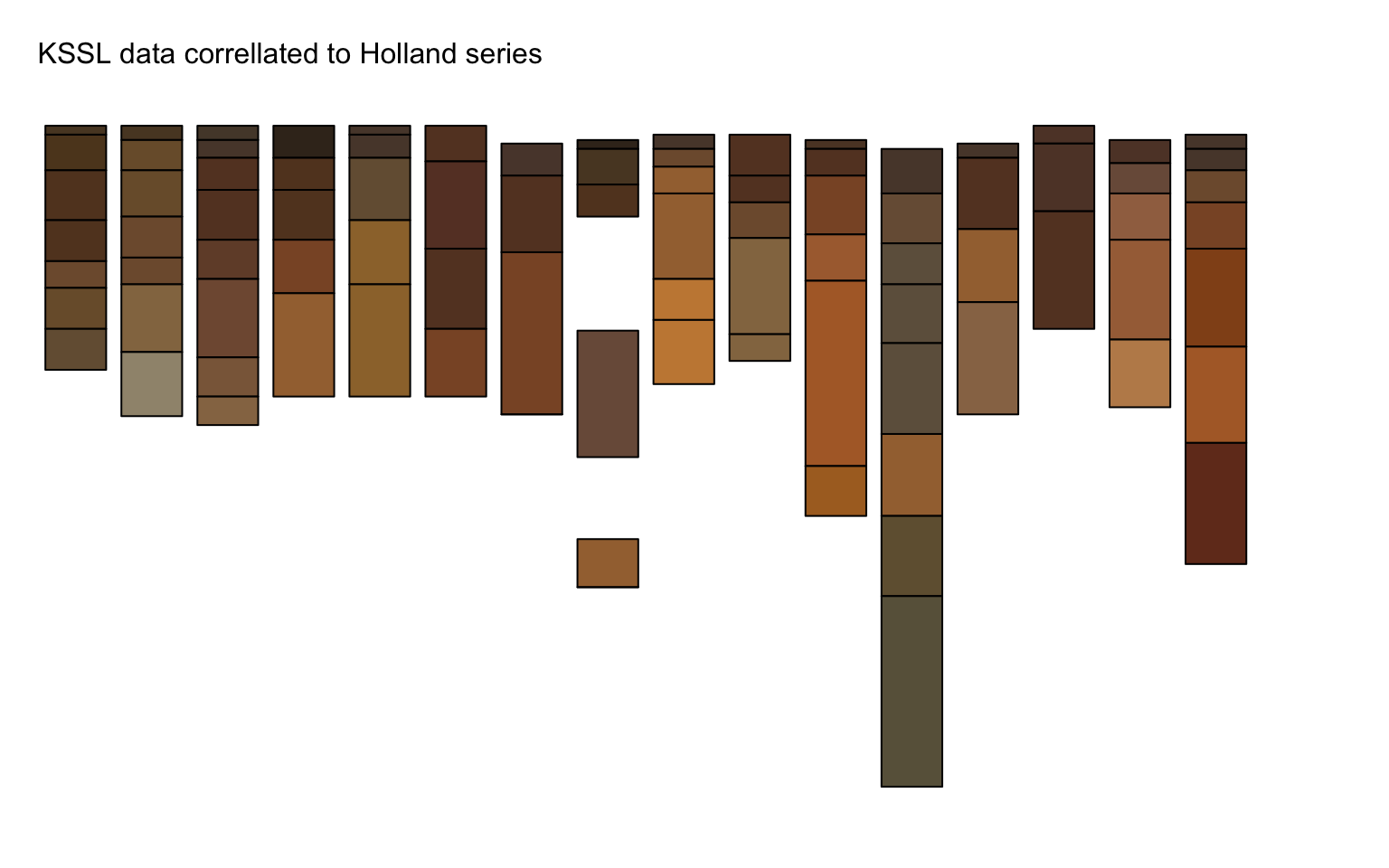A soil color / transition probability (TP) matrix experiment. Transition probabilities are computed in horizon depth order, starting from the top of each profile. The TP matrix can be used to investigate likely sequences and end-points, or, to simulate sequences using Markov chains.

Use pedon data associated with the Holland soil series.

``````library(aqp)
library(soilDB)
library(sharpshootR)
library(igraph)
library(plyr)
library(reshape2)
library(markovchain)
library(RColorBrewer)
library(scales)

# get lab / morphologic data
x <- fetchKSSL(series='holland', returnMorphologicData = TRUE)

# extract pedons into SoilProfileCollection
s <- x\$SPC

# extract horizon data from SPC
h <- horizons(s)

# simplify color data: 1 row / horizon, from morphologic data tables
x.colors <- simplifyColorData(x\$morph\$phcolor, id.var = 'labsampnum', wt='colorpct')

# merge color data into SPC
h <- join(h, x.colors, by='labsampnum', type='left', match='first')

# remove horizons that are missing moist colors
h <- subset(h, h\$m_hue != '' & ! is.na(h\$m_hue) & ! is.na(h\$m_value) & ! is.na(h\$m_chroma))

# re-assemble Munsell color notation for moist color
h\$color <- paste0(h\$m_hue, ' ', h\$m_value, '/', h\$m_chroma)

# pack horizon data back into SPC
horizons(s) <- h``````

Moist color changes with depth.

``````par(mar=c(0,0,2,0))
plot(s, color='moist_soil_color', print.id=FALSE, name='', plot.depth.axis=FALSE, width=0.4)
mtext('KSSL data correllated to Holland series', at=0.5, adj = 0)``````Compute depth-wise transition probability matrix for moist colors. Visualize as a graph organized by communities.

``````# remove profile #8
idx <- (1:length(s))[-8]
s <- s[idx, ]

# generate TP matrix from horizon moist colors
tp <- hzTransitionProbabilities(s, name="color", loopTerminalStates = FALSE)

# greate graph object
par(mar = c(1, 1, 1, 1))
g <- plotSoilRelationGraph(tp, graph.mode = "directed", vertex.scaling.factor=2, edge.arrow.size = 0.5, edge.scaling.factor = 2.5, vertex.label.cex = 0.75, vertex.label.family = "sans")``````